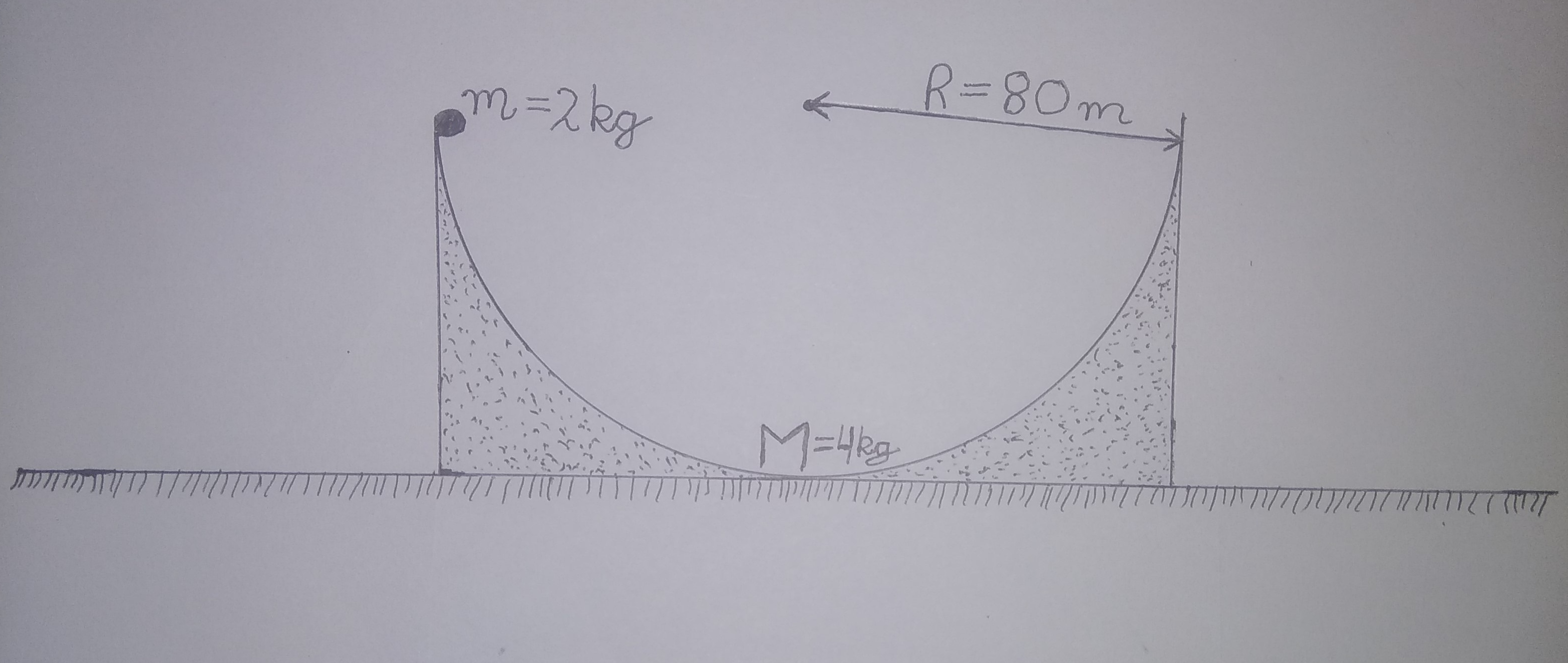# Moving WedgeA wedge of mass $M = 4 \text{ kg}$ and a particle of mass $m = 2\text{ kg}$ are arranged as shown in above figure. The wedge is semicircular, has radius $R = 80\text{ m}$ and is $\text{free to move}$. Find $\text{time period} = T$ of above system in $\text{seconds}$.

Enter answer as $T$.

# Details and Assumptions

• All surfaces are $\color{#3D99F6}{\text{smooth}}$

• Take acceleration due to gravity $\color{#3D99F6}{g = 10m/s^2}$.

• Wedge is $\color{#3D99F6}{\text{free to move}}$

Inspiration Aniket Sanghi

All of my problems are original

×